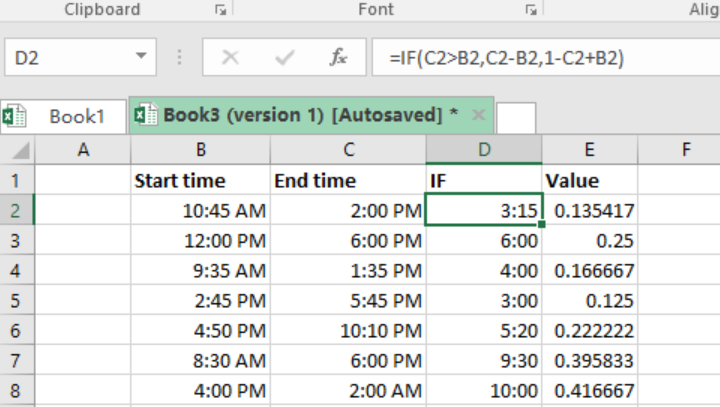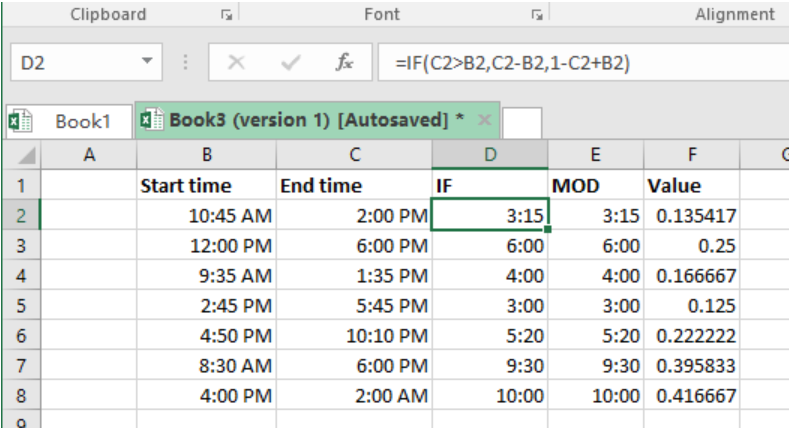Get instant live expert help with Excel or Google Sheets“My Excelchat expert helped me in less than 20 minutes, saving me what would have been 5 hours of work!”

#### Post your problem and you'll get expert help in seconds

Your message must be at least 40 characters
Our professional experts are available now. Your privacy is guaranteed.

# Calculate number of hours between two times

Most of the time we want to manage our free time. Excel allows us to calculate number of hours between two times, a concept that is needed when we want to plan our time well. This post will provide you with a guide on how to calculate number of hours between two times.Figure 1: How to find number of hours between two times

## General syntax of the formula

`=end - start`

## Understanding the formula

It is easy to get the duration between two times using excel. All we need to know is the start and end times. Once we have these two, we can proceed as follows to get the time difference between the two times;

• Specify the start and end times
• Subtract start time from end time.

When start time and end time are in same day, then it becomes easy and straightforward to calculate the time difference.

For instance, if our start time is 11:00 AM and end time is 3:00 PM, we can simply subtract start time from end time as shown below;

`=3:00 PM-11:00 AM`

`=0.166667 hours`

## But what if time crosses a day?

Note that excel treats one day as 1 representing 24 hours. This is why we have the value column in the above example, which represents the fractional values of 1.

Take an example where the start time value is larger than the end time value, o simply put, the end time is the next day after the start time. If we use our formula above, we shall get a negative value, which will be displayed as a string of harsh characters, #####

But we can modify our formula to accommodate the time difference when time crosses a day boundary. The modified formula will look as the one below;

`=1- start + end`

This can be seen in figure 2 row 8 below;Figure 2: Example of how to find number of hours between two times

## The MOD function alternative

In figure 2 above, you must have noticed the MOD column. The MOD function is fundamental in this process as well. It helps us simplify the formula as shown below;

`=MOD (end – start,1)`

The MOD function removes the negative problem by simply flipping values to the required positive values. When using this, you don’t have to put much emphasis on the conditional IF function.

## Instant Connection to an Expert through our Excelchat Service

Most of the time, the problem you will need to solve will be more complex than a simple application of a formula or function. If you want to save hours of research and frustration, try our live Excelchat service! Our Excel Experts are available 24/7 to answer any Excel question you may have. We guarantee a connection within 30 seconds and a customized solution within 20 minutes.

### Did this post not answer your question? Get a solution from connecting with the expert.Another blog reader asked this question today on Excelchat:## Subscribe to Excelchat.coAnother blog reader asked this question today on Excelchat: